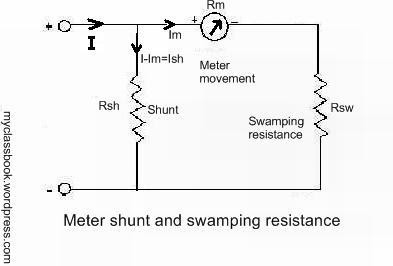# Effects of temperature changes on Ammeter

## Effects of temperature changes in Ammeters:

This post provides an information about effects of temperature changes in ammeters.

Errors due to temperature changes in ammeters can be eliminated by using the same material for both shunt and moving coil and kept at the same temperature. But in practice this method is not suitable because temperature of both parts (shunt and moving coil) does not changes at the same rate. If we use same material like copper, there is one more disadvantaging of copper that they are likely to be bulky as the resistivity of the copper is small.

So to avoid these difficulties, there is one another method in which we use swamping resistance.The arrangement of this method is as shown in the figure. In this method we use a resistance of material having negligible temperature coefficient like mangnin and this resistance is called as ‘swamping resistance’. Its resistance is equal to 20 to 30 times the resistance of the coil used in ammeter. The swamping resistance is connected in series with the coil and shunt of mangnin is connected across this combination as shown in figure. Since copper forms a small fraction of the series combination, the proportion in which the currents would divide between the meter and the shunt would not change appreciably with the change in the temperature.

In this manner the effects of change in temperature on ammeters can be minimized.